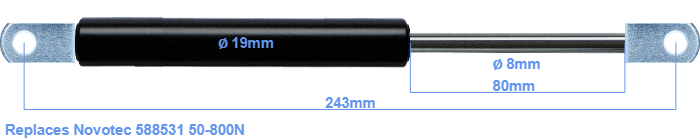# Replacement for Novotec 588531 50-800N

\$30.08

Replacement gas spring for the Novotec 588531 50-800 Newton. The eye has a thickness of 10mm and a hole diameter of 8.1mm. Brand: Stabilus Industry Line.

Please note:
• The thickness of the eye of this replacement is 10mm instead of 5mm.
• The diameter of the tube of this replacement gas spring is 19mm instead of 18mm.
•  Force Choose an option50 Newton60 Newton80 Newton100 Newton120 Newton140 Newton150 Newton160 Newton180 Newton200 Newton220 Newton240 Newton250 Newton260 Newton280 Newton300 Newton320 Newton340 Newton350 Newton360 Newton380 Newton400 Newton420 Newton440 Newton450 Newton460 Newton480 Newton500 Newton520 Newton540 Newton550 Newton560 Newton580 Newton600 Newton620 Newton640 Newton650 Newton660 Newton680 Newton700 Newton720 Newton740 Newton750 Newton760 Newton780 Newton800 NewtonClearThe diameter of the black cylinder of this gas spring is 19 millimeter. The diameter of the rod is 8 millimeter. The chrome part is called the rod. It has a length of 80 mm. The total length of this replacement is 243 millimeter. This is the total distance between the centers of the two mounting parts. Without the mounting parts this gas spring is 205 mm long (thread to thread). The force of this replacement is 50-800 Newton. This is not an original gas spring produced by Novotec, but it can replace a Novotec gas spring. This gas spring was produced by Stabilus subsidiary Hahn with the brand Stabilus Industry Line. The gas spring can be used without problems as a replacement, because the dimensions and force nearly equal.